Chapter Notes: Probability

# Probability Chapter Notes - Mathematics (Maths) Class 10

 Table of contentsBasic TermsWhat is Probability?Different Types of EventsExamples## Basic Terms

• Probability: Probability is a concept which numerically measures degree of certainty of the occurrence of events.
• Experiment: An operation which can produce some well-defined outcomes is called an experiment.
• Random Experiment: An experiment in which all possible outcomes are known.
• Trial: means performing a random experiment
• Outcomes: An outcome is what comes out as a result of a random test or trial.

## What is Probability?

Probability is a way to understand how likely something is to happen using numbers. It's like measuring how uncertain an event's outcome is. We use a scale from 0 to 1, where 0 means it can't happen at all, and 1 means it's certain to happen. You can also think of this scale as percentages, where 0% means impossible and 100% means certain.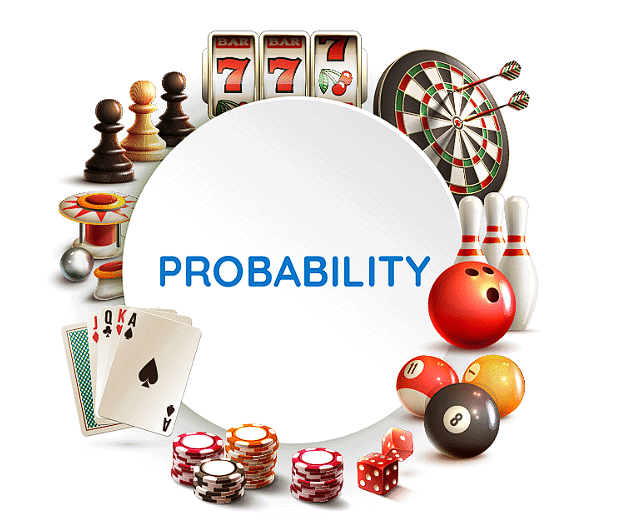For example , if we flip a coin, the chance of getting heads is the same as the chance of getting tails, both 1/2 or 50%. When we add these chances, we get a total probability of 1.

## Different Types of Events

Event and Outcome:

An outcome is the result of something random, like rolling a dice and getting a certain number. An event is a collection of outcomes, like rolling a dice and hoping for a number less than five.

Experimental Probability:

This is when we do an experiment many times and see how often an event happens. For example, if a shopkeeper sells 15 balls and 6 of them are red, the probability of selling a red ball on the next day is 6/15 or 2/5.

Theoretical Probability:

Here, we assume all outcomes are equally likely. If you pick a ball from a basket with 5 red and 7 blue balls, the probability of getting a red ball is 5/12.

Elementary Event:

This is when there's only one possible outcome, like flipping a coin and getting heads or tails.

Sum of Probabilities:

If you add up the probabilities of all possible outcomes, it should equal 1. Like when you flip a coin, the probability of heads plus the probability of tails equals 1.

Impossible and Sure Events:

An impossible event has a probability of 0, like rolling a 7 on a regular dice. A sure event has a probability of 1, like getting a number less than 7 when you throw a dice.

Range of Probability:

Probabilities range between 0 and 1, where 0 means impossible and 1 means certain.

Geometric Probability:

This is about calculating the chance of hitting a specific area on a shape. It involves dividing the desired area by the total area.

Complementary Events:

These are two outcomes that cover all possibilities, like getting heads or tails when flipping a coin. If one event is the opposite of the other, they're complementary.

Example 1 : Find the probability of getting a head when a coin is tossed once. Also

find the probability of getting a tail.

Solution : In the experiment of tossing a coin once, the number of possible outcomes

is two — Head (H) and Tail (T). Let E be the event ‘getting a head’. The number of

outcomes favourable to E, (i.e., of getting a head) is 1. Therefore,

Number of outcomes favourable to E

Number of all possible outcomes

=1/2

Similarly, if F is the event ‘getting a tail’, then

P(F) = P(tail) =1/2

### Probability of Occurrence of an Event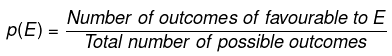For example, what is the probability of the getting head, when we toss the coin.
Possible Outcomes when we toss a coin: Head or Tail
So, we have two possible outcomes.
So, number of possible outcomes =2
So, we have only one favorable outcome
Probability is given as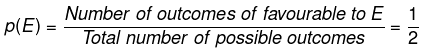## Examples

### (a) Tossing A Coin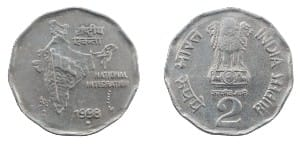If we toss a coin, it would be head or tail, only two outcomes.

### (b) Tossing A Dice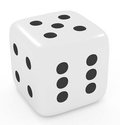A dice has six outcomes, numbering 1 to 6.

### Example of Experiment: Deck of Cards

A deck of playing cards contains 52 Cards.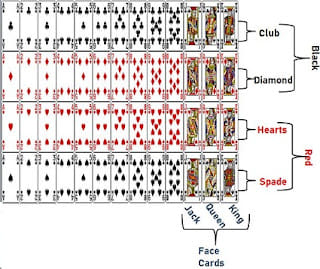If we pick any card from the deck, the outcome will be any one card out of these 52.
There are 13 sets of same type of card. The 4 types of cards are Club, diamond, hearts and Spade.
Heart and spade are red in color, while club and diamond are black in color.

So, there are 13 club, 13 spade, total 26 Black Cards; and 13 hearts, 13 spade total of 26 red cards.

There are 3 face cards in each set.
Jack, Queen and king.
So, there are 3 face cards on each of the 4 sets. So, there are 12 face cards.
Also, we can say there are 6 black face cards, and 6 red face cards.
Apart from face cards, other cards are A, and cards numbering 2 to 10.

### Example of Experiment: Tossing two dice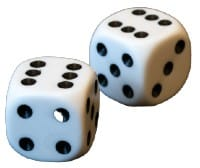Now, there will be 6 outcomes of each dice, multiplied we will get 6×6 = 62 =36 Outcomes. They are: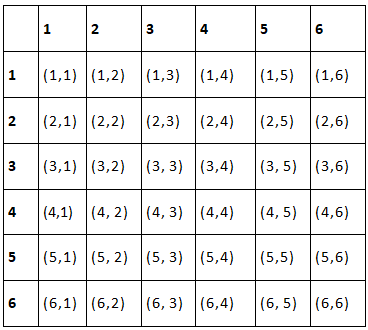The document Probability Chapter Notes | Mathematics (Maths) Class 10 is a part of the Class 10 Course Mathematics (Maths) Class 10.
All you need of Class 10 at this link: Class 10

## Mathematics (Maths) Class 10

115 videos|478 docs|129 tests

## FAQs on Probability Chapter Notes - Mathematics (Maths) Class 10

 1. What is probability in mathematics?Ans. Probability is a branch of mathematics that deals with the measurement of uncertainty. It is used to quantify the likelihood of an event occurring, ranging from 0 (impossible) to 1 (certain). Probability helps us make predictions and informed decisions based on the available information.
 2. How is probability calculated?Ans. Probability is calculated by dividing the number of favorable outcomes by the total number of possible outcomes. The formula for probability is: Probability = Number of favorable outcomes / Total number of possible outcomes This formula is used in various probability problems to determine the chances of an event happening.
 3. What is the difference between theoretical probability and experimental probability?Ans. Theoretical probability is based on mathematical calculations and assumes that all outcomes are equally likely. It is determined by the number of favorable outcomes divided by the total number of possible outcomes. On the other hand, experimental probability is based on actual observations or experiments. It involves collecting data through observations or trials and calculating the probability based on the observed results. Experimental probability can be used when the theoretical probability is not known or when the situation involves real-life events.
 4. How is probability related to statistics?Ans. Probability and statistics are closely related fields. Probability provides the foundation for statistical analysis by quantifying uncertainty and randomness. It helps in making predictions and analyzing data. Statistics, on the other hand, involves collecting, organizing, analyzing, and interpreting data to make informed decisions or draw conclusions. Probability plays a crucial role in statistical analysis, as it helps in determining the likelihood of events or outcomes based on data.
 5. What are some real-life applications of probability?Ans. Probability has various real-life applications. Some examples include: 1. Weather forecasting: Probability is used to predict the likelihood of rain, snow, or other weather conditions. 2. Insurance: Probability is used to calculate insurance premiums by assessing the likelihood of specific events, such as accidents or damage. 3. Gambling: Probability is essential in games of chance, such as card games or lotteries, to determine the likelihood of winning. 4. Risk assessment: Probability is used to assess the probability of certain risks or events occurring, such as in financial investments or project management. 5. Medical research: Probability is used in clinical trials and medical research to assess the effectiveness of treatments and determine the likelihood of certain outcomes.

## Mathematics (Maths) Class 10

115 videos|478 docs|129 testsExplore Courses for Class 10 examSignup to see your scores go up within 7 days! Learn & Practice with 1000+ FREE Notes, Videos & Tests.
10M+ students study on EduRev
Track your progress, build streaks, highlight & save important lessons and more!
Related Searches

,

,

,

,

,

,

,

,

,

,

,

,

,

,

,

,

,

,

,

,

,

;